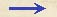Discorsi Propositions 3/14-pr-07 Discorsi Proposition3/14-pr-07{308} PROPOSITIO XIV. {308} PROPOSITION XIV Altitudinis atque sublimitates semiparabolarum, quarum amplitudines aequales futurae sunt, per singulos elevationis gradus reperire. To find for each degree of elevation the altitudes and sublimities of parabolas of constant amplitude. Haec omnia facili negotio consequemur: posita enim semiparabolae amplitudine partium semper 10000, medietas tangentis cuiuslibet gradus elevationis altitudinem exhibet.Ut, exempli gratia, semiparabolae, cuius elevatio sit gr. 30, amplitudo vero, et ponitur, partium 10000, altitudo erit 2887; tanta enim est proxime medietas tangentis.Inventa autem altitudine, sublimitatem eliciemus tali pacto.Cum demonstratum sit, dimidiam amplitudinem semiparabolae mediam esse proportionalem inter altitudinem et sublimitatem, {10} sitque altitudo iam reperta, medietas vero amplitudinis semper eadem, partium sit 5000, si huius quadratum per altitudinem datam diviserimus, sublimitas quaesita exurget.Ut, in exemplo, altitudo reperta fuit 2887; quadratum partium 5000 est 25000000; quod divisum per 2887, dat 8659 proxime pro sublimitate quaesita. The problem is easily solved. For if we assume a constant amplitude of 10000, then half the tangent at any angle of elevation will be the altitude. Thus, to illustrate, a parabola having an angle of elevation of 30° and an amplitude of 10000, will have an altitude of 2887, which is approximately one-half the tangent. And now the altitude having been found, the sublimity is derived as follows. (Condition 3/05-pr-02-cor) Since it has been proved that half the amplitude of a semi-parabola is the mean proportional between the altitude and sublimity, and since the altitude has already been found, and since the semi-amplitude is a constant, namely 5000, it follows that if we divide the square of the semi-amplitude by the altitude we shall obtain the sublimity sought. Thus in our example the altitude was found to be 2887: the square of 5000 is 25,000,000, which divided by 2887 gives the approximate value of the sublimity, namely 8659.Discorsi Propositions 3/14-pr-07 Discorsi Proposition3/14-pr-07